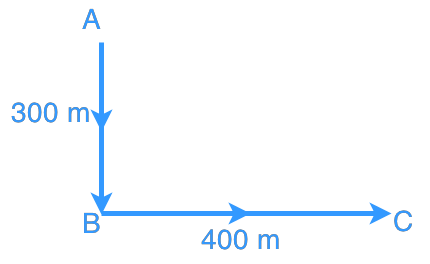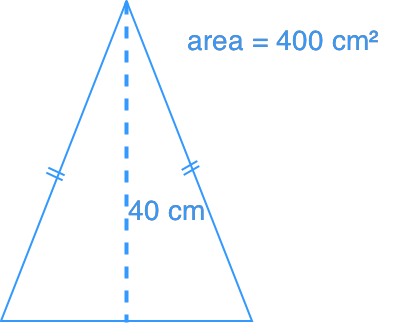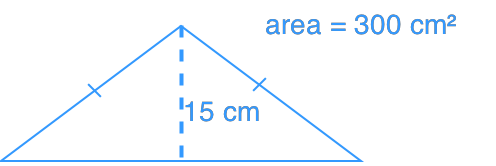# Applications of pythagorean theorem

### Applications of pythagorean theorem

We will need to apply Pythagorean Theorem often in our daily life. In this lesson, we will focus on tackling some Pythagorean Theorem word problems.

#### Lessons

• Introduction
a)

i) What is Pythagorean Theorem?

ii) How to use Pythagorean Theorem to find sides of a triangle?

b)
What are Pythagorean Triples?

• 1.
An aeroplane flew from point A to point B, and then to Point C. How far is the plane from point A? Refer to the diagram below.• 2.
A 2.5 m long ladder is put against a wall. If the base of the ladder is 1.25 m away from the wall, what is the length from the ground to the point where the top of ladder touches the wall?

• 3.
Which triangle has a longer perimeter?
Triangle ATriangle B